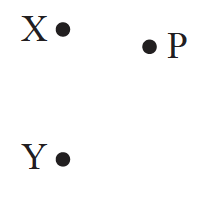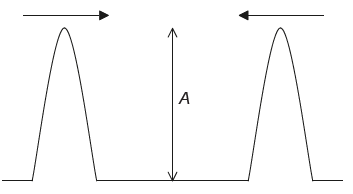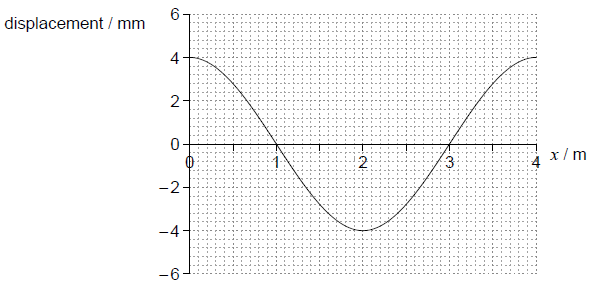1. Two waves meet at a point in space. Which of the following properties always add together?
2. Which graph correctly shows how the acceleration,

a of a particle undergoing SHM varies with its displacement,  x  from its equilibrium position?

3. A transverse travelling wave has an amplitude

x_0 and wavelength λ. What is the minimum distance between a crest and a trough measured in the direction of energy propagation?

4. A point source emits sound waves of amplitude A. The sound intensity at a distance d from the source is /. What is the sound intensity at a distance 0.5d from the source when the source emits waves of amplitude 2A?
5. In which of the following regions of the electromagnetic spectrum is radiation of wavelength 600 nm located?
6. A student stands a distance L from a wall and claps her hands. Immediately on hearing the reflection from the wall she claps her hands again. She continues to do this, so that successive claps and the sound of reflected claps coincide. The frequency at which she claps her hands is f. What is the speed of sound in air?
7. A particle is displaced from rest and released at time t = 0. It performs simple harmonic motion (SHM). Which graph shows the variation with time of the kinetic energy

Ek of the particle?

8. What is the phase difference, in rad, between the centre of a compression and the centre of a rarefaction for a longitudinal travelling wave?
9. A wave on a string travels to the right as shown. The frequency of the wave is

f At time t=0, a small marker on the string is in the position shown.

What is the position of the marker at t=1/(4f)?10. Electromagnetic waves
11. Electromagnetic waves
12. The frequency of the first harmonic standing wave in a pipe that is open at both ends is 200 Hz. What is the frequency of the first harmonic in a pipe of the same length that is open at one end and closed at the other?
13. Two travelling waves are moving through a medium. The diagram shows, for a point in the medium, the variation with time t of the displacement d of each of the waves.For the instant when = 2.0 ms, what is the phase difference between the waves and what is the resultant displacement of the waves?14. An orchestra playing on boat X can be heard by tourists on boat Y, which is situated out of sight of boat X around a headland.The sound from X can be heard on Y due to

15. A wave pulse travels along a light string which is attached to a frictionless ring. The ring can move freely up and down a vertical rod.What is the shape of the wave pulse after reflection?

16. A pipe of length L has two open ends. Another pipe of length L′ has one open end and one closed end.

The frequency of the first harmonic of both pipes is the same. What is (L')/L?

17. Two identical waves of wavelength λ leave two sources in phase. The waves meet and superpose after travelling different distances. Which path difference will result in destructive interference?
18. Which of the following gives regions of the electromagnetic spectrum in the order of decreasing frequency?
19. Which of the following is a value of wavelength that is found in the visible region of the electromagnetic spectrum?
20. For a body undergoing simple harmonic motion the velocity and acceleration are
21. In simple harmonic oscillations which two quantities always have opposite directions?
22. The power emitted as electromagnetic radiation by the Sun is approximately

4×1026 W. The radius of the orbit of Mars around the Sun is approximately 2×1011 m. What is the best estimate for the power incident on an area of 1 m2 at the radius of Mars’ orbit?

23. The intensity of radiation from a star at the surface of one of its planets is /. The distance between the star and the planet is d.

What is the intensity at the surface of another planet which is a distance d/4 from the star?

24. Waves emitted from sources X and Y have equal wavelengths and are initially in phase. The waves interfere destructively at point P, where the path difference is 0.60m.What is a possible value for the wavelength of the waves?

25. A girl in a stationary boat observes that 10 wave crests pass the boat every minute. What is the period of the water waves?
26. What statement about X-rays and ultraviolet radiation is correct?
27. A pipe of fixed length is closed at one end. What is28. The refractive index for light travelling from medium X to medium Y is

4/3 . The refractive index for light travelling from medium Y to medium Z is 3/5 is the refractive index for light travelling from medium X to medium Z?

29. A body undergoes one oscillation of simple harmonic motion (shm). What is correct for the direction of the acceleration of the body and the direction of its velocity?
30. The diagram shows an interference pattern produced by two sources that oscillate on the surface of a liquid.Which of the distances shown in the diagram corresponds to one fringe width of the interference pattern?

31. Two sound waves from a point source on the ground travel through the ground to a detector. The speed of one wave is

7.5 km s–1, the speed of the other wave is 5.0 km s–1. The waves arrive at the detector 15 s apart. What is the distance from the point source to the detector?

32. A pair of slits in a double slit experiment are illuminated with monochromatic light of wavelength 480 nm. The slits are separated by 1.0 mm. What is the separation of the fringes when observed at a distance of 2.0 m from the slits?
33. What is true about the acceleration of a particle that is oscillating with simple harmonic motion (SHM)?
34. A ray of light is incident on a boundary between glass and air.Which of the following is the refractive index of glass?

35. A system that is subject to a restoring force oscillates about an equilibrium position.

For the motion to be simple harmonic, the restoring force must be proportional to

36. What are the changes in the speed and in the wavelength of monochromatic light when the light passes from water to air?37. A sound wave has a wavelength of 0.20 m. What is the phase difference between two points along the wave which are 0.85 m apart?
38. Monochromatic light travels from air into water. Which of the following describes the changes in wavelength and speed?39. One end of a horizontal string is fixed to a wall. A transverse pulse moves along the string as shown.Which of the following statements are correct for the reflected pulse compared to the forward pulse?

I.     It moves more slowly.

II.     It has less energy.

III.     It is inverted.

40. A first-harmonic standing wave is formed on a vertical string of length 3.0 m using a vibration generator. The boundary conditions for this string are that it is fixed at one boundary and free at the other boundary.The generator vibrates at a frequency of 300 Hz.

What is the speed of the wave on the string?

41. Two wave pulses, each of amplitude A, approach each other. They then superpose before continuing in their original directions. What is the total amplitude during superposition and the amplitudes of the individual pulses after superposition?42. The graph shows the variation with distance x of the displacement of the particles of a medium in which a longitudinal wave is travelling from left to right. Displacements to the right of equilibrium positions are positive.Which point is at the centre of a compression?

43. The graph shows how the velocity

v of an object undergoing simple harmonic motion varies with time t for one complete period of oscillation.Which of the following sketch graphs best shows how the total energy E of the object varies with t?

44. A high solid wall separates two gardens X and Y. Music from a loudspeaker in X can be heard in Y even though X cannot be seen from Y. The music can be heard in Y due to
45. What region of the electromagnetic spectrum includes waves of wavelength

5 ×10–8 m?